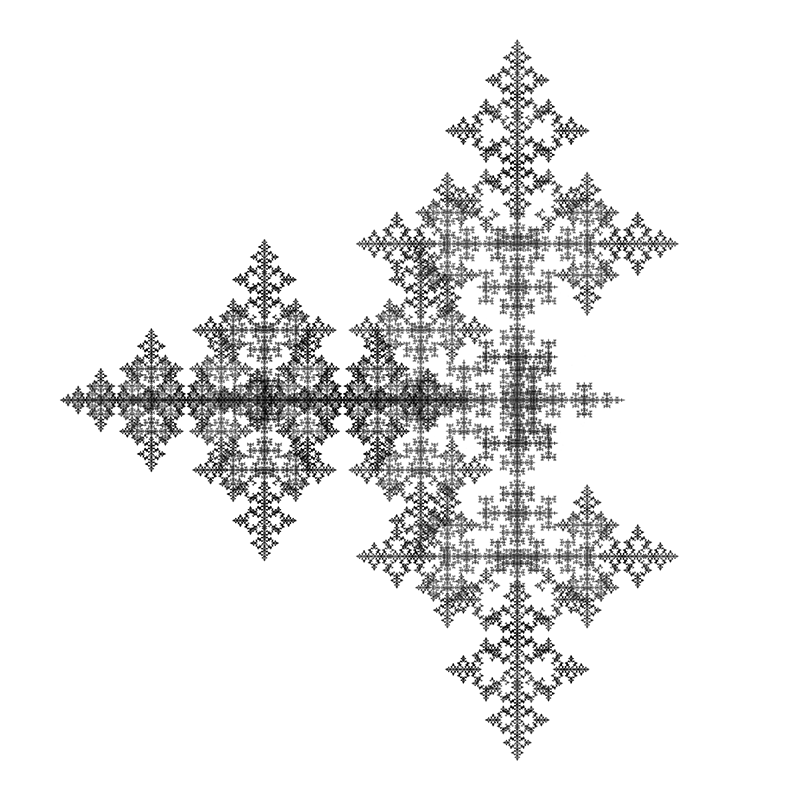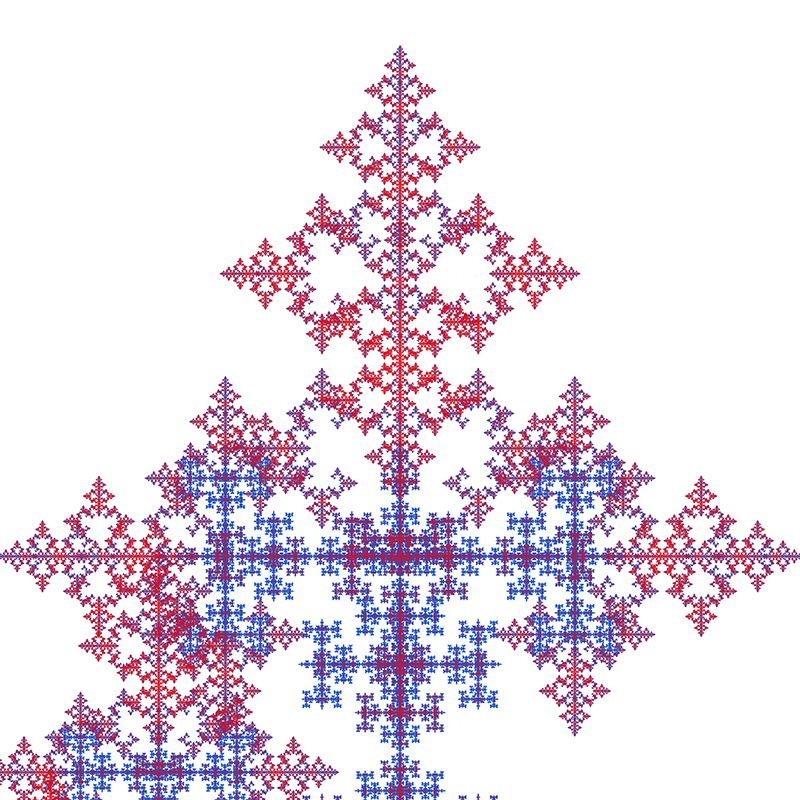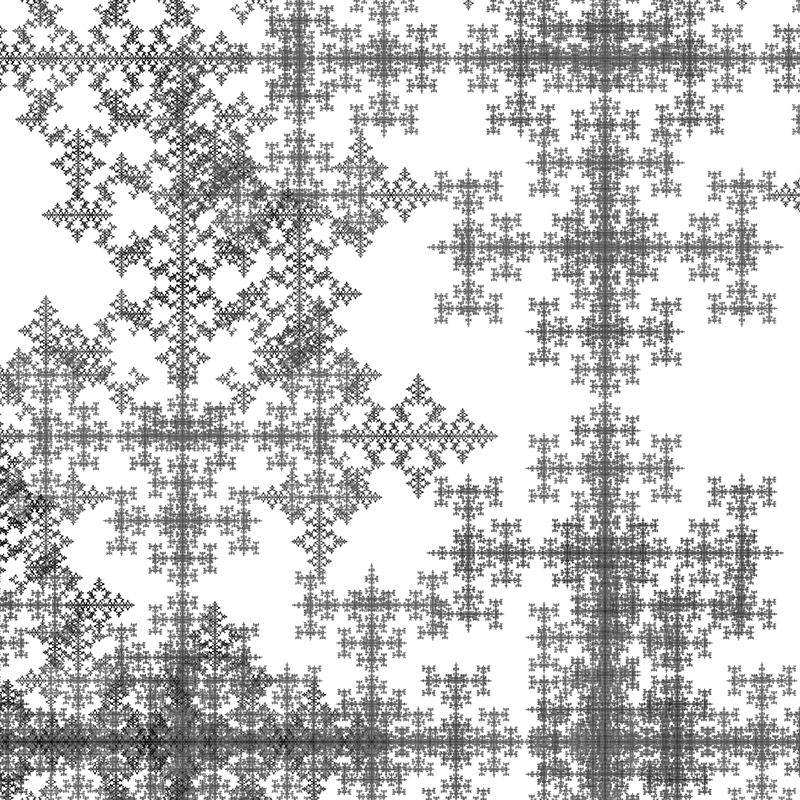# Five center factorization for the Mandelbrot set

Inspired by Roger Bagula
Paul Bourke
Original June 2002, images updated January 2018In doing work on fuzzy logic and tiling I have been looking at group theory again. The simple groups are the cyclic rotational groups on the unit circle that I use in my Sierpinski set work. I will develop a factoring method of powered Mandelbrots:

1) z = zs + c

I will , then, show a five center factorization for the Mandelbrot set. I will actually develop this for the equations of the type:

2) z = zn - c

The reason for this will become clear as I derive my results. Let's start with:

3) z = z5 - c

It is a four lobe figure. They are at right angles to each other. It is a natural for a rotation of 90 degrees in group terms:

4) z = z * (z - a1) * (z - a2) * (z - a3) * (z - a4)

5) a12 = a2

6) a13 = a3

7) a14 = a4 = 1

The solution for this is:

8) a1 = i and 4) becomes:

9) z = z * (z - i) * (z + 1) * (z + i) * (z - 1)
z = z * (z2 + 1) * (z2 - 1)
z = z * (z4 - 1)
z = z5 - z

So how do we get 3) out of this? We let :

10) ac1 = i * (c / z)0.25 and the equation is:

11) z = z * (z - i * (c / z)0.25) * (z + 1 * (c / z)0.25) * (z + i * (c / z)0.25) * (z - 1 * (c / z)0.25)

Which following through the steps in 9) above gives 3). These are the fixed points that result in the four lobes. Now I have experimentally found five attractors for the Mandelbrot set. If we substitute in:

12) z -> z0.4 and

13) ac1 -> i * (c / z)0.1 into 11) we get:

14) z = z2 - c

So we have the conjugate to the Mandelbrot set with five attractor points! Lets do 9) backwards from the positive instead of the negative:

15) z = z5 + z ( a Newtons algorithm type)
z = z * (zs4 + 1)
z = z * (z2 + i) * (z2 - i)
z = z * (z + i * sqr(i)) * (z - i * sqr(i)) * (z + sqr(i)) * (z - sqr(i))

So in all cases above just adding the Sqr(i) term makes the Mandelbrots come out. So lets put it altogether in 11):

16) z1 = z0.4

17) c1 = (c / z)0.1

18) z = z1 * (z1 + i * sqr(i) * c1) * (z1 - i * sqr(i) * c1) * (z1 + sqr(i) * c1) * (z1 - sqr(i) * c1)

That equation gives a factorization of the limiting attracting fixed points of the Mandelbrot set. It has taken me years to get where I can do this. The relationship of groups and fractals has been a theme in my work from the beginning. In the special unitary groups there is a pairing relationship of SU(2) and SU(5) . The moment of inertia of a sphere is:

19) I = m * rs2 * (2/5)

So that the power of 2/5 here isn't totally surprising. In my Sierpinski set dimensional spectra work, I had estimated that the dimensionality of the Mandelbrot set was:

20) hd = log(5) / log(2)

That idea came from the five attractor points and the observed limiting similarity scaling effects. I don't have an actual numerical solution for these limiting attractor points yet, but at least I have a better method than before! The actual nature of these equations suggests that the Mandelbrot set is not Sierpinski in nature, but Eisenstein fraction! It is the reverse sign and zero center that give it that characteristic. Since the Julia sets of the Mandelbrot set have the same form, the same factors apply. The observation of a phase between three points and five points in experimentation, indicates a degeneracy between these attractors happens when the constant is right. The question of what:

21) z = z0.4 + c

Might have as a shape also seems important from the similarity type argument. Knowledge is a long term process with fits and starts and mistakes. This article using group theory seems to answer some long term problems, but more questions are raised as a result. If I had given up several years ago when I got negative feed back on my ideas about the Mandelbrot set, I would never have gotten this far. The strange negative directional onesidedness of the Mandelbrot set seems to be due to two of the centers moving to the same side in the limiting case in a way much like the golden mean. An attractor set like:

22) Set = {0, -gm1, -gm2, i, -i} : gm1 = (1 + sqr(5)) / 2: gm2 = gm1 - 1

Would have the right kind of properties for what we observe.